##### Physics I For Dummies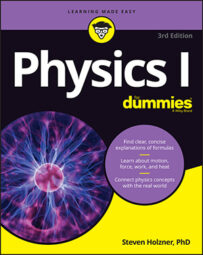You can use physics to calculate the kinetic energy of an object. When you start pushing or pulling a stationary object with a constant force, it starts to move if the force you exert is greater than the net forces resisting the movement, such as friction and gravity. If the object starts to move at some speed, it will acquire kinetic energy. Kinetic energy is the energy an object has because of its motion. Energy is the ability to do work.

So how do you calculate kinetic energy?

A force acting on an object that undergoes a displacement does work on the object. If this force is a net force that accelerates the object (according to Newton’s second law), then the velocity changes due to the acceleration. The change in velocity means that there is a change in the kinetic energy of the object.

The change in kinetic energy of the object is equal to the work done by the net force acting on it. This is a very important principle called the work-energy theorem. After you know how work relates to kinetic energy, you’re ready to take a look at how kinetic energy relates to the speed and mass of the object.

The equation to find kinetic energy, KE, is the following, where m is mass and v is velocity: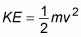Using a little math, you can show that work is also equal to (1/2)mv2. Say, for example, that you apply a force to a model airplane in order to get it flying and that the plane is accelerating. Here’s the equation for net force:

F = ma

The work done on the plane, which becomes its kinetic energy, equals the following: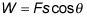Net force F equals mass times acceleration. Assume that you’re pushing in the same direction that the plane is going; in this case, cos 0 degrees = 1, so

W = Fs = mas

Assuming constant acceleration, you can tie this equation to the final and original velocity of the object. Use the equation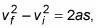where vf equals final velocity and vi equals initial velocity. Solving for a gives you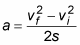If you plug this value of a into the equation for work, W = mas, you get the following: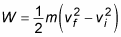If the initial velocity is zero, you get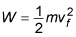This is the work that you put into accelerating the model plane — that is, into the plane’s motion — and that work becomes the plane’s kinetic energy, KE: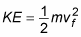This is just the work-energy theorem stated as an equation.

You normally use the kinetic energy equation to find the kinetic energy of an object when you know its mass and velocity. Say, for example, that you’re at a firing range and you fire a 10-gram bullet with a velocity of 600 meters/second at a target. What’s the bullet’s kinetic energy? Using the equation to find kinetic energy, you simply plug in the numbers, remembering to convert from grams to kilograms first to keep the system of units consistent throughout the equation: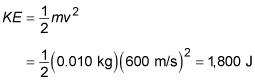The bullet has 1,800 joules of energy, which is a lot of energy to pack into a 10-gram bullet.